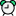# Hiremee Logarithms Questions with Answers

Question 1Time: 00:00:00
If log(k2 - 4k + 5) = 0, then the value of k is -0

0

2

2

1

1

3

3

Once you attempt the question then PrepInsta explanation will be displayed.Start

Question 2Time: 00:00:00
log (1 + 2 + 3) = log 1 + log 2 + log 3log10 10 = 1

log10 10 = 1

log (2 + 3) = log (2 x 3)

log (2 + 3) = log (2 x 3)

log10 1 = 0

log10 1 = 0

log (1 + 2 + 3) = log 1 + log 2 + log 3

log (1 + 2 + 3) = log 1 + log 2 + log 3

Once you attempt the question then PrepInsta explanation will be displayed.

log(1+2+3)=log(1×2×3)=log 6 Log(2+3)=log(2×3)=log 6 //log(m+n)=log mnStart

Question 3Time: 00:00:00
If log 2 = 0.3010 and log 3 = 0.4771, the value of log5 512 is:2.870

2.870

2.967

2.967

3.876

3.876

3.912

3.912

Once you attempt the question then PrepInsta explanation will be displayed.Start

Question 4Time: 00:00:00
If log 27 = 1.431, then the value of log 9 is:0.934

0.934

0.945

0.945

0.954

0.954

0.958

0.958

Once you attempt the question then PrepInsta explanation will be displayed.Start

Question 5Time: 00:00:00
If loga/b + logb/a = log (a + b), then:a + b = 1

a + b = 1

a - b = 1

a - b = 1

a = b

a = b

apow2 - bpow2 = 1

apow2 - bpow2 = 1

Once you attempt the question then PrepInsta explanation will be displayed.Start

Question 6Time: 00:00:00
The value of1/log3 60 + 1/log4 60 +1/log5 60is:0

0

1

1

5

5

60

60

Once you attempt the question then PrepInsta explanation will be displayed.